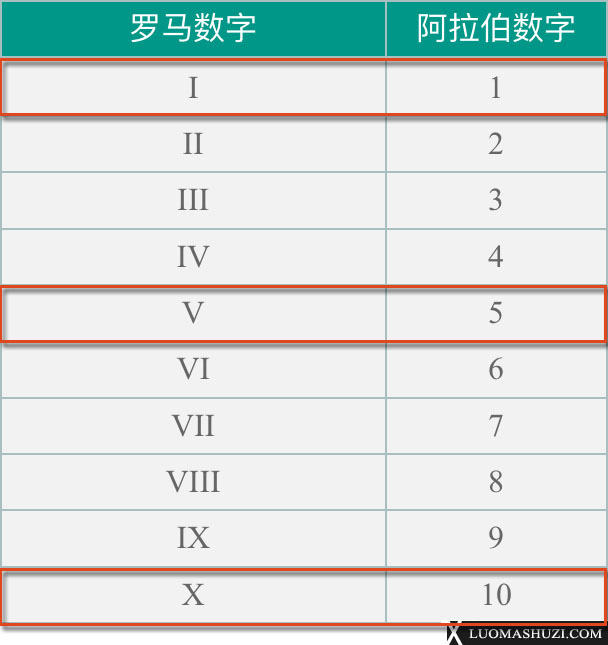#### 罗马数字I到X(阿拉伯数字1到10)的记忆方法

##### 发布于：2018-07-23 03:17:01I V X
1 5 10

1. 因为罗马数字I表示阿拉伯数字1，那么在I的基础上，其右侧再写一个I，那么就是：II = 1+1 = 2。同理再在右侧写1个I，那么就是：III = 1+1+1 = 3
2. 因为同一个符号一般情况下，不能连续使用超过三次，所以第4个数就不能再继续右侧写1个I了，而是在V的左侧写1个I，即：IV = 5-1 = 4

3. 按照第2条的规则，那么我们就可以很顺利的写出：VI = 5+1 = 6VII = 5+1+1 = 7VIII = 5+1+1+1=8；
4. 根据第2条的原理，那么阿拉伯数字9就应该写成： IX = 10-1 = 9

I 1 1
II 2 1+1 = 2
III 3 1+1+1 = 3
IV 4 5-1 = 4
V 5 5
VI 6 5+1 = 6
VII 7 5+1+1 = 7
VIII 8 5+1+1+1 = 8
IX 9 10-1 = 9
X 10 10